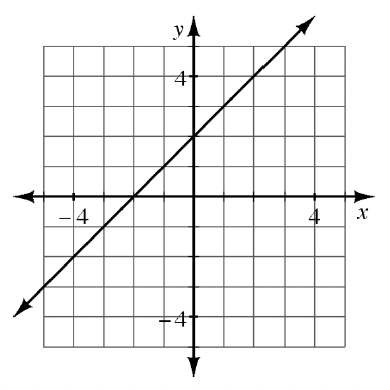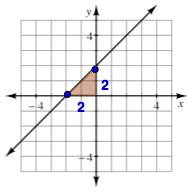### Home > AC > Chapter 8 > Lesson 8.1.3 > Problem8-31

8-31.Find the equation of a line perpendicular to the one graphed below that passes through the point $(6, 2)$.

Find the slope of the line at right by making a slope triangle.

$\text{Slope}=\frac{2}{2}=1$Use the $y = mx + b$ form of a line to start writing your equation.
Use the opposite reciprocal of the slope you found in order to make the line perpendicular to the one graphed at right.

$y=-1x+b$

Substitute the given point into the equation to solve for $b$.

$\begin{array}{l} 2 = -1\left(6\right) + b \\ 2 = -6 + b \\ 8 = b \end{array}$

Substitute $y$ and $x$ back into their original places in the equation, and you have your equation for a line perpendicular to the one that is graphed at right.